##### Signals and Systems For DummiesThe block diagram of a system that uses a digital filter to remove an SNOI and retain the SOI is shown. This system assumes that the signals originate in continuous time and, after filtering, are returned to the continuous-time domain.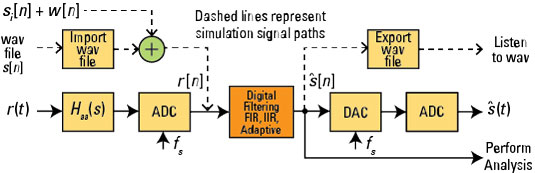Credit: Illustration by Mark Wickert, PhD

The analog-to-digital converter (ADC) and digital-to-analog converter (DAC) interfaces represent how a real-time system is configured; but later, when simulating this system, you’ll use wav files to process prerecorded speech (the dashed lines).

The received signal, r(t), is of the form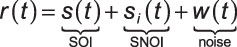From this point forward, assume that noise is negligible and drop w(t) from consideration.

The heart of the system is the digital filter block that sits between the ADC and DAC. The SOI is speech of nominal bandwidth 4 kHz, and the SNOI is one or more sinusoidal tones somewhere on the 0- to 4-kHz band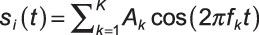Consider the finite impulse response (FIR), infinite impulse response (IIR) and adaptive FIR filtering options shown.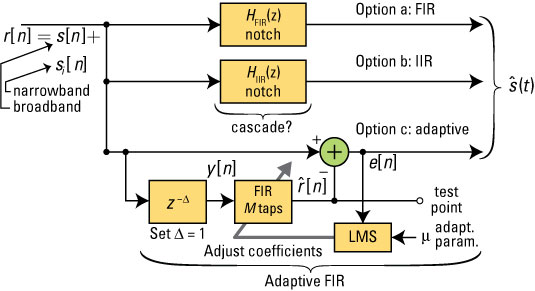Credit: Illustration by Mark Wickert, PhD

The FIR notch filter has system function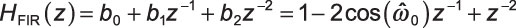which is a pair of conjugate zeros at angle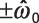relative to the positive real axis in the z-plane. The IIR notch filter adds a pair of conjugate poles at radius r behind the FIR zeros that are on the unit circle: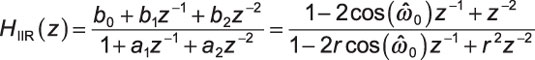The pole-zero plots for these two filters are shown. Here, the plots are created with functions in the Python module ssd.py. For the IIR: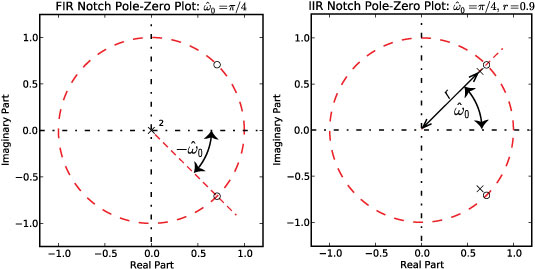Credit: Illustration by Mark Wickert, PhD
```In [<b>528</b>]: b,a = ssd.fir_iir_notch(1000,8000,0)
In [<b>529</b>]: ssd.zplane(b,a,1.2)
Out[<b>529</b>]: (2, 0)
In [<b>532</b>]: b,a = ssd.fir_iir_notch(1000,8000,0.9)
In [<b>533</b>]: ssd.zplane(b,a,1.2)
Out[<b>533</b>]: (2, 2)```

The FIR notch has a single design parameter, while the IIR notch, also has the pole radius, r. Given the sampling rate, fs Hz, and the interference tone frequency, fk Hz, set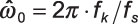If more than one interfering tone is present, create a cascade of notch filters — one for each interfering tone, with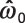set accordingly.

With two IIR notch filters in cascade, the convolution theorem for z-transforms says that the overall system function is a fourth-order IIR filter: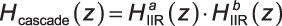You can find the coefficients through convolution of second-order numerator and denominator coefficient sets.

The adaptive filter is more exotic. This filter is an example of a time-varying linear system. Don’t worry about fully absorbing the math behind this filtering system/algorithm right now. The intent here is to provide you with a general sense about its operation, inspiring you to study this topic further at some time in the future.

A FIR filter is at the core of this system, but the coefficients aren’t fixed: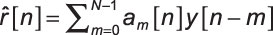has N coefficients am[n] that are updated (changed) following the output of each new signal sample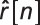m in am[n] is the filter coefficient index, and n denotes the time update. The least mean squares (LMS) adaptation algorithm is responsible for adjusting the filter coefficients in such a way that the average of the squared error (mean squared error [MSE]) at time n,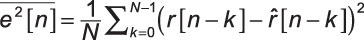is minimized.

In this application, you have the adaptive filter configured to perform interference cancellation. The error output e[n] is an estimate of the SOI,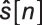The output of the FIR filter, denotedis also an estimate of the SNOI,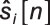Unlike the FIR and IIR notch filters, the adaptive filter adjusts its coefficients to form a passband response (shaped as a band-pass) at the location of each interfering tone. Giving the filter a large number of taps (or degrees of freedom) allows it to form multiple passbands if needed. It does this on its own, without any prior information!

Upon convergence of the LMS, the output tends to contain only the SNOI, which is one or more sinusoids. The error output is the (SOI + SNOI) – SNOI = SOI. An estimate of the SOI is exactly what you want, but this filter is more complex. Also, if the SOI by chance contains steady tones, the adaptive filter does its best to remove them. Eliminating desired tones from your SOI is likely a showstopper.

For each sample n, the LMS algorithm performs three steps:

1. Calculates the following where n is the time index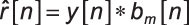The filter coefficients utilized at time n are am[n], for m = 0, 1, . . ., M.

2. Forms the error sequence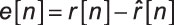3. Updates the filter coefficients, using a stochastic (instantaneous) gradient to estimate the direction of steepest descent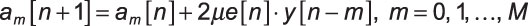The parameter μ is known as the convergence parameter. If μ is too small, then filter convergence is slow; but if it’s too large, then the algorithm becomes unstable. An approximate upper bound on μ is 1 / [(M + 1)Pr], where Pr is the power r[n]. As initial conditions, set all the filter coefficients to 0.

For a two-tap FIR, you can view the steepest descent as it’s shown. Each update of the LMS algorithm moves you on average toward the bottom of the error surface, thus minimizing the MSE.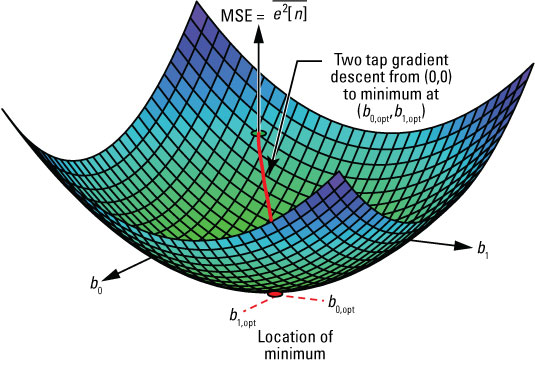Credit: Illustration by Mark Wickert, PhD